# Linear Equations 1 1 1 2 1 3

• Slides: 20Linear Equations • • • 1. 1 1. 2 1. 3 1. 4 1. 5 1. 7 System of linear Equations Row Reduction and Echelon Forms Vector Equations 向量方程 The Matrix Equation Ax = b 矩阵方程 Solution Sets of Linear Systems Linear Independence 线性无关• Solution b = 3 a 1+ 2 a 2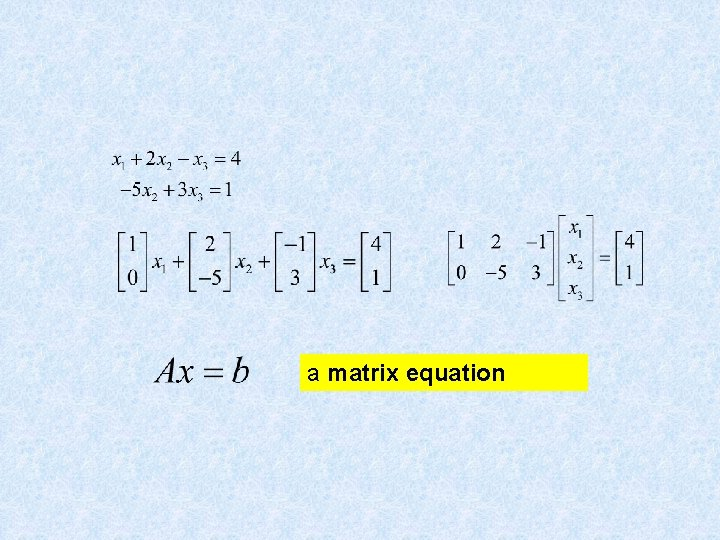a matrix equation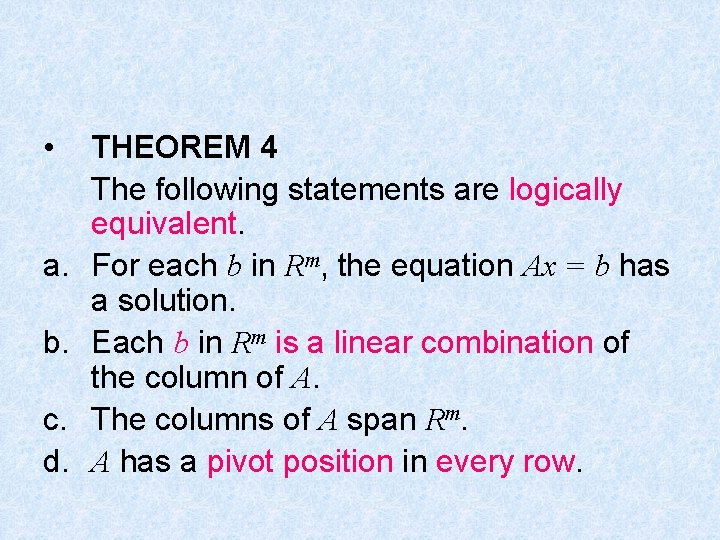• a. b. c. d. THEOREM 4 The following statements are logically equivalent. For each b in Rm, the equation Ax = b has a solution. Each b in Rm is a linear combination of the column of A. The columns of A span Rm. A has a pivot position in every row.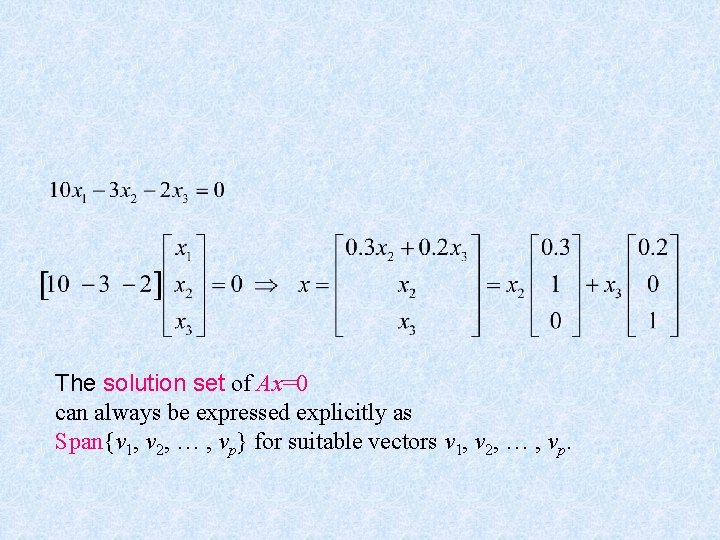The solution set of Ax=0 can always be expressed explicitly as Span{v 1, v 2, … , vp} for suitable vectors v 1, v 2, … , vp.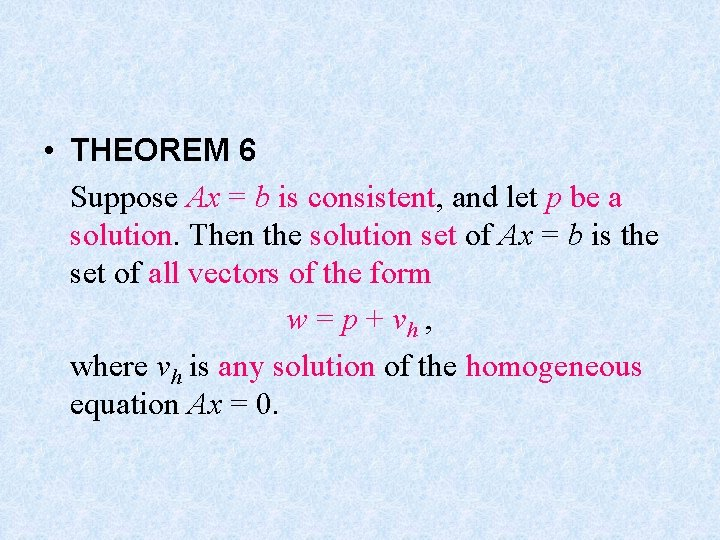• THEOREM 6 Suppose Ax = b is consistent, and let p be a solution. Then the solution set of Ax = b is the set of all vectors of the form w = p + vh , where vh is any solution of the homogeneous equation Ax = 0.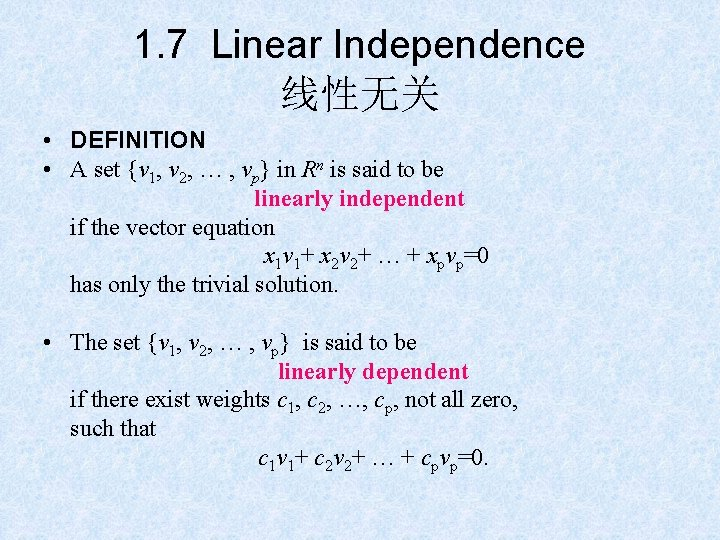1. 7 Linear Independence 线性无关 • DEFINITION • A set {v 1, v 2, … , vp} in Rn is said to be linearly independent if the vector equation x 1 v 1+ x 2 v 2+ … + xpvp=0 has only the trivial solution. • The set {v 1, v 2, … , vp} is said to be linearly dependent if there exist weights c 1, c 2, …, cp, not all zero, such that c 1 v 1+ c 2 v 2+ … + cpvp=0.• A set is linearly dependent if and only if it is not linearly independent. • 不是“线性独立” • 没有“线性有关”这个词，应该是“线性相关”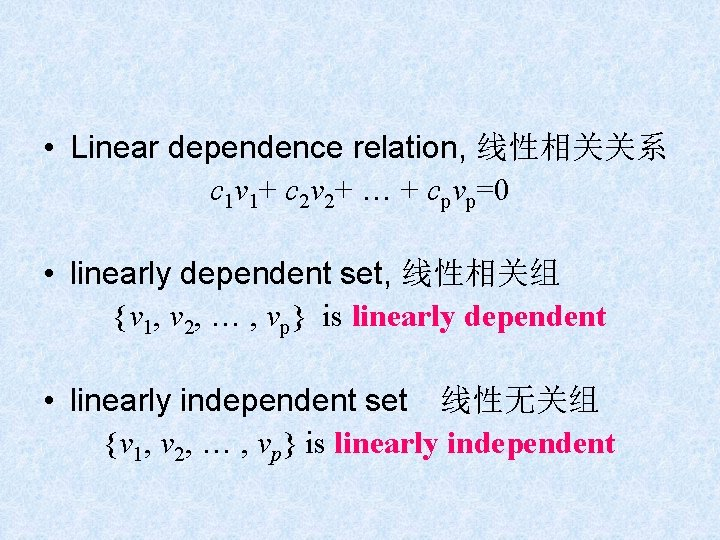• Linear dependence relation, 线性相关关系 c 1 v 1+ c 2 v 2+ … + cpvp=0 • linearly dependent set, 线性相关组 {v 1, v 2, … , vp} is linearly dependent • linearly independent set　线性无关组 {v 1, v 2, … , vp} is linearly independent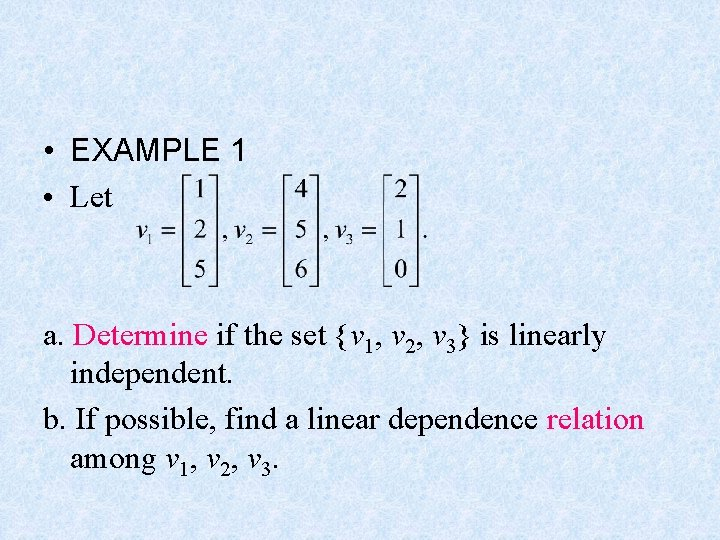• EXAMPLE 1 • Let a. Determine if the set {v 1, v 2, v 3} is linearly independent. b. If possible, find a linear dependence relation among v 1, v 2, v 3.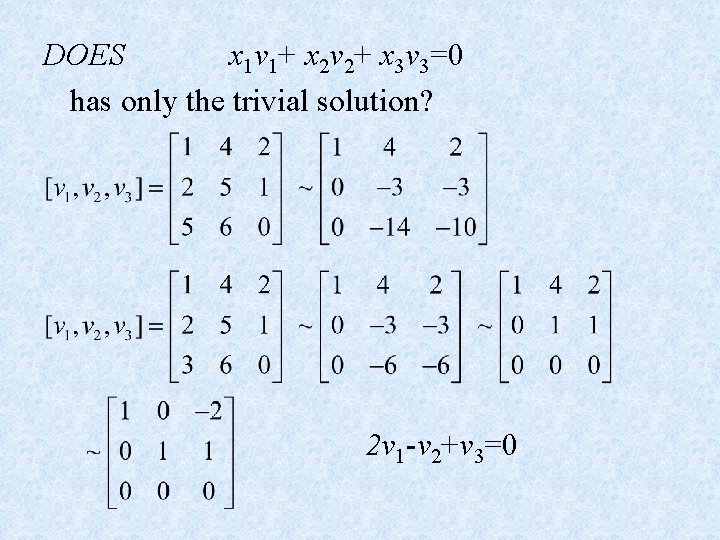DOES x 1 v 1+ x 2 v 2+ x 3 v 3=0 has only the trivial solution? 2 v 1 -v 2+v 3=0Linear Independence of Matrix Columns The columns of a matrix A are linearly independent if and only if the equation Ax=0 has only the trivial solution. Ax=0 A 1 x 1+ A 2 x 2+…+ Anxn+=0• EXAMPLE 2 Determine if the column of the matrix are linearly independent.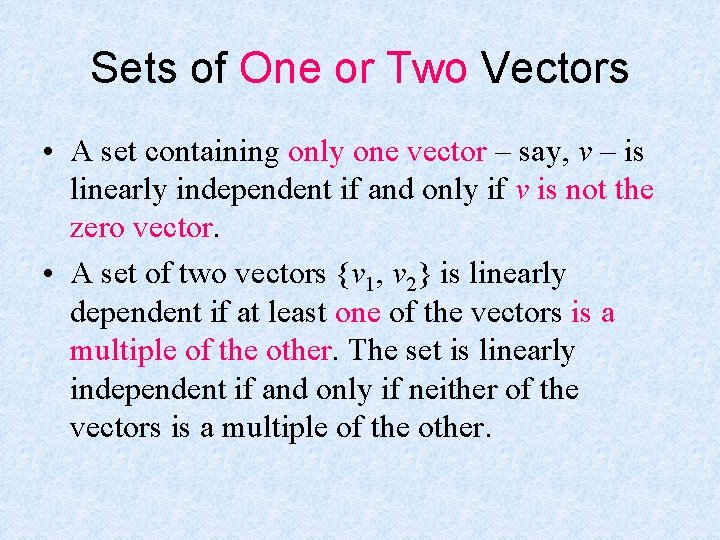Sets of One or Two Vectors • A set containing only one vector – say, v – is linearly independent if and only if v is not the zero vector. • A set of two vectors {v 1, v 2} is linearly dependent if at least one of the vectors is a multiple of the other. The set is linearly independent if and only if neither of the vectors is a multiple of the other.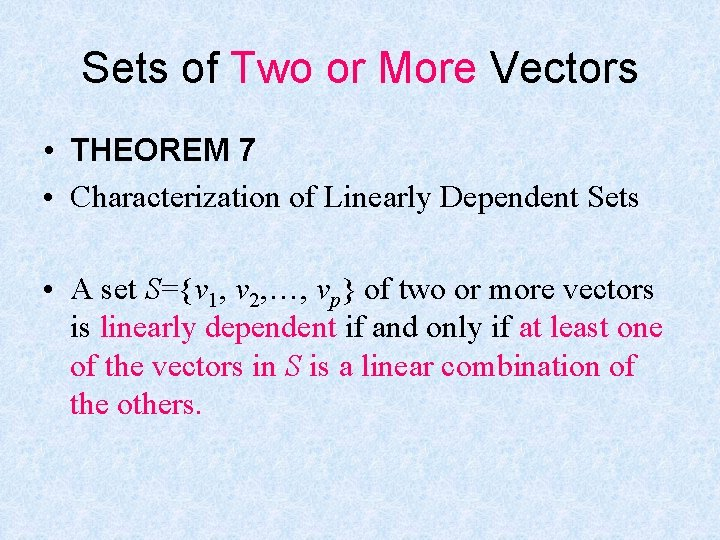Sets of Two or More Vectors • THEOREM 7 • Characterization of Linearly Dependent Sets • A set S={v 1, v 2, …, vp} of two or more vectors is linearly dependent if and only if at least one of the vectors in S is a linear combination of the others.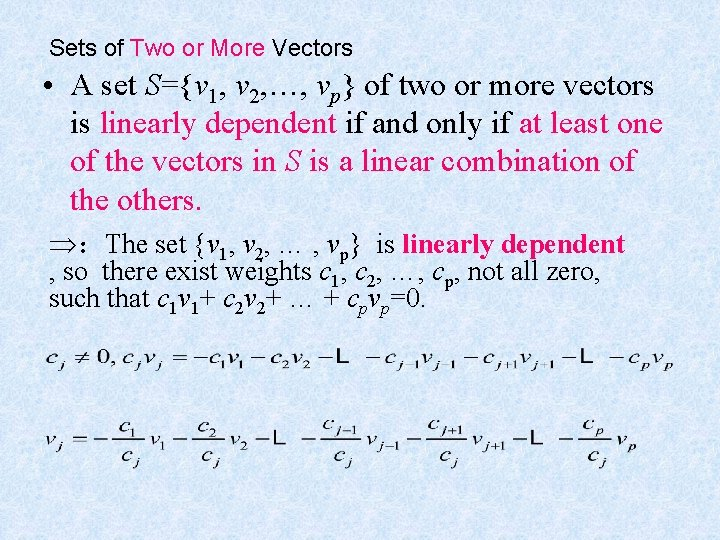Sets of Two or More Vectors • A set S={v 1, v 2, …, vp} of two or more vectors is linearly dependent if and only if at least one of the vectors in S is a linear combination of the others. ：The set {v 1, v 2, … , vp} is linearly dependent , so there exist weights c 1, c 2, …, cp, not all zero, such that c 1 v 1+ c 2 v 2+ … + cpvp=0.Sets of Two or More Vectors • THEOREM 7 • Characterization of Linearly Dependent Sets • A set S={v 1, v 2, …, vp} of two or more vectors is linearly dependent if and only if at least one of the vectors in S is a linear combination of the others. If S is linearly dependent and v 1 0, then some vj is a linear combination of the preceding vectors, v 1, v 2, …, vj-1.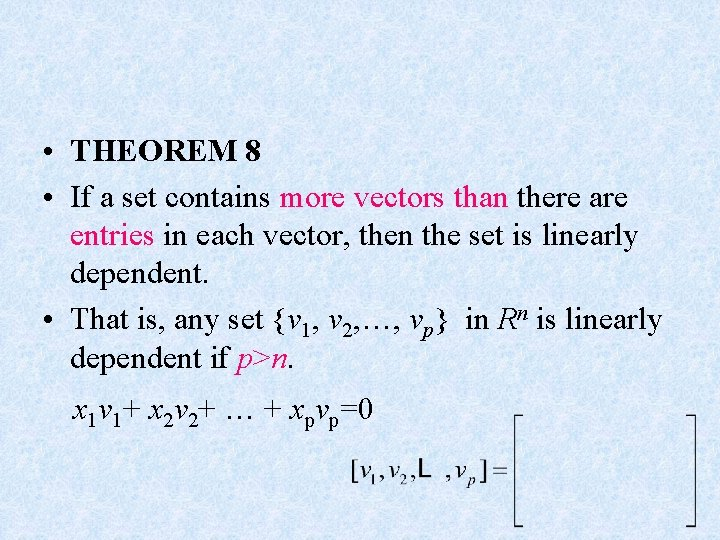• THEOREM 8 • If a set contains more vectors than there are entries in each vector, then the set is linearly dependent. • That is, any set {v 1, v 2, …, vp} in Rn is linearly dependent if p>n. x 1 v 1+ x 2 v 2+ … + xpvp=0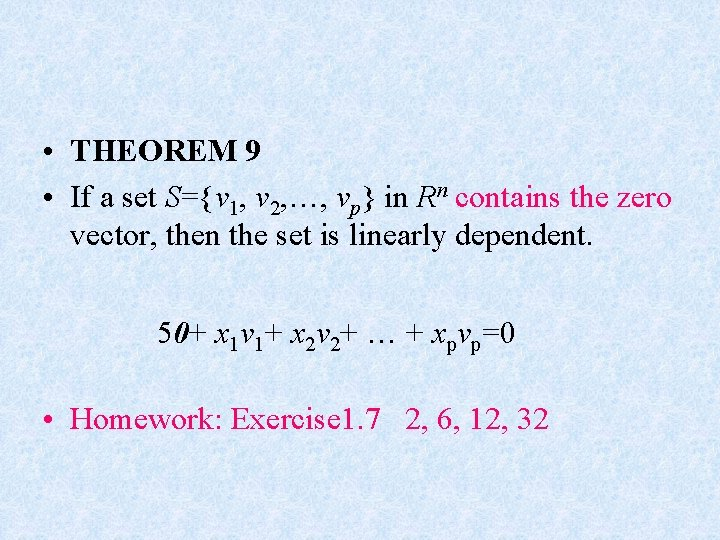• THEOREM 9 • If a set S={v 1, v 2, …, vp} in Rn contains the zero vector, then the set is linearly dependent. 50+ x 1 v 1+ x 2 v 2+ … + xpvp=0 • Homework: Exercise 1. 7 2, 6, 12, 32